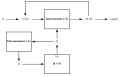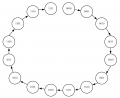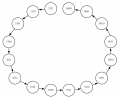# Implement 4-bit Incrementer with two 74LS163 + 74LS74A + XNOR gate(s) + AND gate(s)

#### veroverobueno

Joined Nov 23, 2019
3
I have been struggling with the following question for a long time.
Use two 74LS163, one 74LS74A (D flip-flop), XNOR gate(s) and AND gate(s) to build a circit that can "add" two given 4-bit binary numbers A and B.

Here is how the circuit computes SUM = A+B (for SUM <= 1111):

1. Load A into a counter.

2. Count up by number of clocks given by B to obtain SUM.

Hint: one 74LS163 will give you the SUM. The other 74LS163 will tell the first one when to stop counting.

You must place A and B into the inputs of the circuit, and initialise the circuit by setting/resetting/loading necessary register(s) using a signal INITIALISE = 0.

-----------------------------------------------

I am very new to this topic so I'm not sure if any of the following is correct.

Here is a simple chart of how I believe the circuit works:State diagram for SUM:State diagram for B:#### veroverobueno

Joined Nov 23, 2019
3
(I just realised that I can't edit what I've already posted)

I wanna add that I know how to use 74LS163, but I am not sure how to construct the entire circuit.

Let's say we're adding B to A. Here is what I've come up with so far:
74LS163 (count):
- counts from 0 to B
- LOAD = combinational circuit to detect when Q = B (turn B into 1111)​
74LS163 (SUM):
- input = A
- ENP = combinational circuit so that when 74LS163 (count)'s output resets to 0, 74LS163 (SUM) will stop counting​
All chips share the same clock.

Unfortunately, I'm not sure if other in/outputs are needed, nor do I know how to include the D flip-flop.